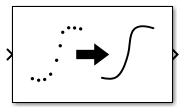# Lowpass Resampler

Convert signal from one sample time to another

Since R2021a

•Libraries:
Mixed-Signal Blockset / Utilities

## Description

The Lowpass Resampler block converts either a fixed-step discrete or a variable-step discrete sample time at its input to a different sample time at its output. To calculate the output sample values, the block uses a lowpass filtering interpolation algorithm. The algorithm minimizes frequency aliasing at the output with respect to an output rise/fall time.

If the output rise/fall time is inherited from a fixed-step discrete input, the cutoff frequency is the Nyquist rate of the input. Otherwise, the cutoff frequency is the Nyquist rate associated with a sample interval obtained by scaling the specified 20%−80% output rise/fall time to a value for 0%−100% rise/fall time.

## Ports

### Input

expand all

Discrete time input signal, specified as fixed-step or variable-step scalar.

Data Types: `single` | `double` | `int8` | `int16` | `int32` | `uint8` | `uint16` | `uint32` | `Boolean`

### Output

expand all

Continuous time output signal, returned as a fixed-step or variable-step scalar.

Data Types: `single` | `double`

## Parameters

expand all

Select to inherit the output rise/fall time from the fixed-step input sample time.

Note

In case of variable step input, there is no rise/fall time to inherit.

20%−80% output rise/fall time, specified as a positive real scalar.

#### Dependencies

To enable this parameter, deselect the Inherit output rise/fall time parameter.

#### Programmatic Use

 Block parameter: `OutputRiseFall` Type: character vector Values: positive real scalar Default: `1e-10`

Number of samples of propagation delay for fixed-step operation, specified as a positive real scalar.

If the Lowpass Resampler block inherits Output rise/fall time in fixed-step mode, the resampler conversion delay is given by NDelay·τ, where NDelay is the Number of samples of delay parameter and τ is the inherited input sample interval.

In the variable-step input mode, the resampler conversion delay is given by 0.6τv when NDelay equals to one, and is (NDelay-0.5)·τv when NDelay is greater than one. τv is 5/3 times the Output rise/fall time parameter.

There is a tradeoff between the delay of the conversion and the suppression of out-of-band numerical artifacts, with greater delay producing better fidelity in band and greater suppression out-of-band.

Note

If you need anti-aliasing filter rejection, set Number of samples of delay to 5 or higher.

#### Dependencies

To enable this parameter, deselect the Inherit output rise/fall time parameter.

#### Programmatic Use

 Block parameter: `NDelay` Type: character vector Values: positive real scalar Default: `1`

Type of the output sample time to be used by downstream blocks, specified as either fixed step discrete or variable step discrete. The output sampling rate must be higher than the input sampling rate. For more information, seeVariable Step Sampling Scheme.

#### Programmatic Use

 Block parameter: `OutputTsType` Type: character vector Values: `Fixed step discrete` | ```Variable step discrete``` Default: `Inherited`

Number of output samples in a single output rise/fall time, specified as a positive real scalar.

#### Dependencies

To enable this parameter, select the option ```Fixed step discrete``` or `Variable step discrete` in the Output sample time parameter.

#### Programmatic Use

 Block parameter: `OutputTsRatio` Type: character vector Values: positive real scalar Default: `5`

Select to enable extra buffer samples. This option is enabled by default.

Number of extra buffer samples needed to support the number of samples of delay, specified as a positive real scalar.

#### Programmatic Use

 Block parameter: `NBuffer` Type: character vector Values: positive real scalar Default: `10`